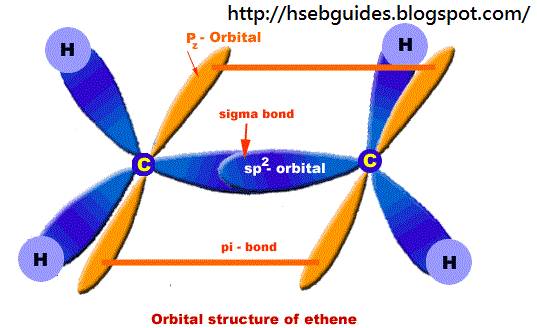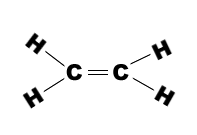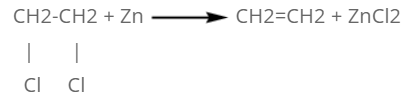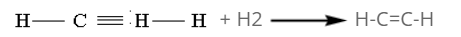# Ethane

Introduction

Molecular formula = C2H4
Empirical formula = CH2
Molecular mass = 28
Empirical formula mass = 14
Homologous series = alkene

Orbital structure of ethane
Composition of ethane:
Ethene molecule consists of two carbon atoms and four H-atoms i.e. CH2=CH2

Nature of hybridization:
In ethene molecule each C-atom is Sp2-hybridized. Due to Sp2-hybridization each C-atom generates three Sp2-hybrid orbitals. In this way there exist six Sp2-hybrid orbital. These Sp2-orbital are arranged in trigonal order and 120o apart. Remaining ‘2pz‘ unhybrid orbital of carbon atom are placed perpendicular to the plane of Sp2-orbitals.

Sigma bond formation:
One Sp2-hybrid orbital of C-atom overlaps with One Sp2-hybrid orbital of second C-atom to produce one sigma bond between two carbon atoms. Remaining two Sp2-orbitals overlap with two H-atom to produce sigma bond. ‘2pz‘ orbitals of two C-atoms are un-hybridized and make parallel overlapping to produce pi-bond as shown below:Bond length:
C=C bond length is 1.34A
C-H bond length is 1.09AMethods of preparation:
By vicinal dihalide:
When vicinal dihalide is heated with zinc dust, two halide atoms are removed from the compound and ethene is formed.By the reduction of ethyne:
Under control conditions, ethyne adds two H-atoms to form ethene in the presence of Nickel(Ni) at 200oC.By the dehydrohalogenation of alkyo halide:
When alkyl halide (ethyl chloride) is treated with alcoholic KOH, b-elimination takes place and ethene is obtained.
CH3-CH2Cl + KOH == CH2=CH2 + KCl + H2O

By the dehydration of alcohol:
When ethyl alcohol is heated with conc.H2SO4 at 170oC, dehydration of alcohol takes place and ethene is formed.
C2H5OH == C2H4 + H2O
Or
Ethyl alcohol may also be converted into ethene by passing vapors of ethyl alcohol over a catalyst (Al2O3, H3PO4/Al2O3). The reaction is carried out at 350C in the presence of Al2O3 and at 250C when H3PO4/Al2O3 is used.
C2H5OH == C2H4 + H2O

Chemical properties
CH2=CH2 + Cl2 == CH2Cl-CH2Cl (1,2-dichloroethane)

CH2=CH2 +Br2 == CH2Br-CH2Br (1,2-dibromoethane)

CH2=CH2 + I2 == CH2I-CH2I (1,2-diiodoethane)

Order of reactivity:
Cl2 > Br2 > I2

CH2=CH2 + H2 == CH3-CH3

CH2=CH2 + H2SO4 == CH3-CH2-HSO4

CH2=CH2 + HOH == CH3-CH2-OH

CH2=CH2 + HCl == CH3-CH2-Cl

CH2=CH2 + HBr == CH3-CH2-Br

CH2=CH2 + HI == CH3+CH2-I

CH2=CH2 + HOCl == OH-CH2-CH2-Cl (Ethane chlorohydrin)

Reaction with clorine water:
When Cl2 is mixed with water, two molecules of acids are formed.
Cl2 + H2Oirreversible_arrow HCl + HOCl
CH2=CH2 + HCl == CH3-CH2-Cl
CH2=CH2 + HOCl == OH- CH2-CH2-Cl

Combustion reaction:
CH2=CH2 + 3O2 == 2CO2 + 2H2O

Oxidation reaction:
When ethene is treated with KMnO4 solution, purple colour of KMnO4 disappears due to deformation of a colorless compound “Ethylene Glycol”.
CH2=CH2 + H2O +[O] == OH-CH2-CH2-OH

Formation of mustard gas:
b,b-Dichloroethyl Sulphide is commonly known as “Mustard gas”. It is a very poisonous gas. It can produce blisters on skin.
2CH2=CH2 + S2Cl2 == Cl-CH2-CH2-S-CH2-CH2-Cl + S

Polymerization:
n(CH2=CH2) == n(-CH2-CH2-)